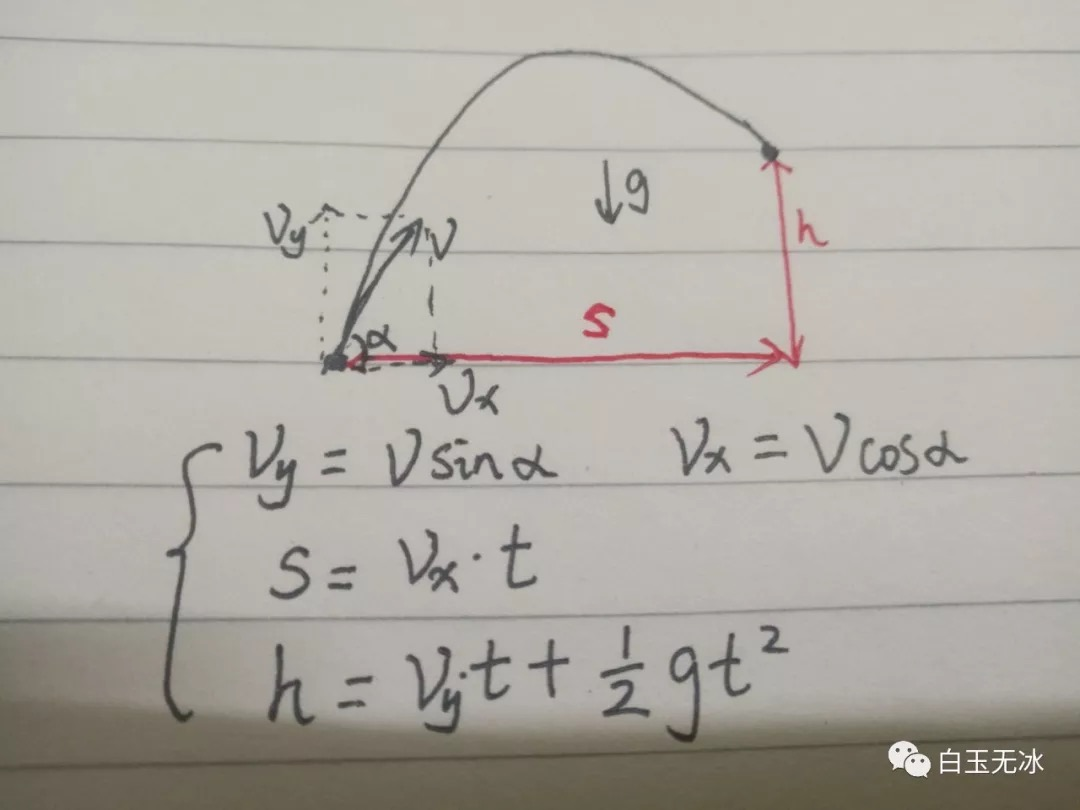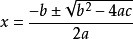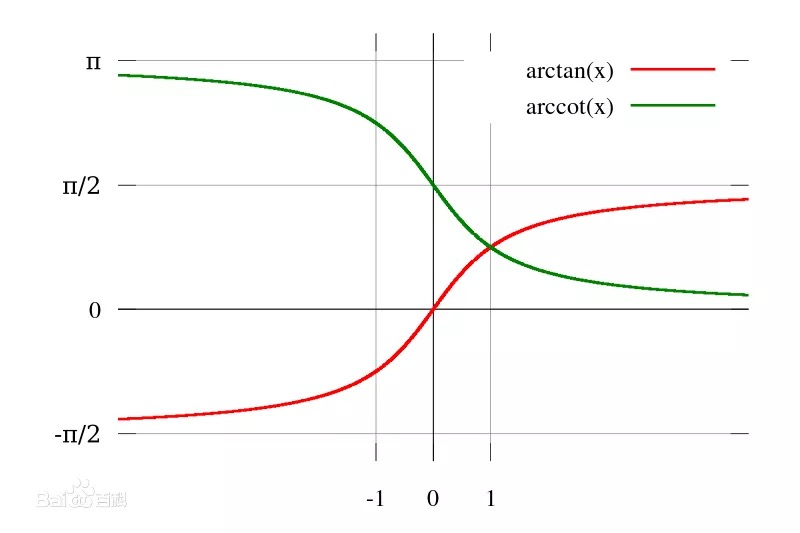# 置顶如何实现高抛或平抛发射？精选热门- 起点发射速度大小

- 重力加速

- 起始点与经过点

- 发射角度```const s = location.x - START_POS.x;
const h = location.y - START_POS.y;
// a*t^2 + b*t + c = 0const a = G * s / ( * V * V);
const b = ;
const c = a - h / s;
const delta = b * b -  * a * c;
if (delta >= ) {
// 一元二次方程求根公式
const t1 = (-b + Math.sqrt(delta)) / ( * a); // 平抛 tan 值
const t2 = (-b - Math.sqrt(delta)) / ( * a); // 高抛 tan 值
// 二、四象限角度要加 180
const alpha1 = Math.atan(t1) + (s <  ? Math.PI : );
const alpha2 = Math.atan(t2) + (s <  ? Math.PI : );
}
```

```const v_x_1 = Math.cos(alpha1) * V;
const v_y_1 = Math.sin(alpha1) * V;
const v_x_2 = Math.cos(alpha2) * V;
const v_y_2 = Math.sin(alpha2) * V;
// 低抛线速度 保存起来this._linearVelocity_1.x = v_x_1;
this._linearVelocity_1.y = v_y_1;
// 高抛线速度 保存起来this._linearVelocity_2.x = v_x_2;
this._linearVelocity_2.y = v_y_2;
```

```// 计算夹角const angle = rigidBody.linearVelocity.clone().signAngle(cc.v2(, ));
rigidBody.node.rotation = angle * 180 / Math.PI;
```

```this.schedule(this.fireArrow, 0.5, cc.macro.REPEAT_FOREVER);
```

```private _index = ;
privatefireArrow) {
const linearVelocity = this.toggle_arrow.isChecked ? this._linearVelocity_2.clone() : this._linearVelocity_1.clone()
const rigidBody_arrow = this._all_arrows[this._index++ % this._all_arrows.length];
rigidBody_arrow.node.setPosition(START_POS);
rigidBody_arrow.linearVelocity = linearVelocity;
}
```

【本文使用图片素材转载自Cocos社区作者：白玉无冰】

1 个评论